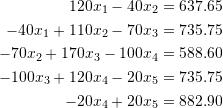### Linear Systems of Equations: Problems

1. Create a recursive function in Mathematica that calculates the determinant and compare it with the built-in determinant function. Test your function on matrices in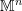for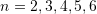, and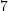.
2. Use Cramer’s rule to solve the linear system of equations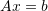defined as: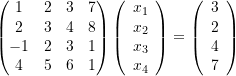3. Use the naive Gauss elimination method to solve the linear system of equationsdefined as:4. Use the Cramer’s rule code, the naive Gauss elimination method code, and the Gauss-Jordan elimination method code given above to test how many “simple” equations the algorithm can solve in less than a minute for each method. You can do so by constructing the identity matrix of dimension(using the command IdentityMatrix[n]) and a vector of values 1 of dimensionand then running the code. The solution to this should be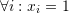. Repeat for higher values of. If you surround your code with the command Timing[] it should give you an indication of how long the computations are taking. Draw the curve between the size of the systemand the timing taken by each method to find the solution. Is the relationship between the time taken to solve the system of equations and the size of the matrix linear or nonlinear?
5. Repeat problem 4, however, in this case, use the commands:
A = RandomReal[{-1, 1}, {n, n}];
b = RandomReal[{-1, 1}, n];


to generate a matrix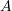that is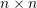with random coefficients between -1 and 1 and a vectorwith random numbers between -1 and 1. Does this affect the results of the time taken to solve the system as a function of the matrix size and the method used?

6. Using random numbers similar to the previous problem, draw the curve showing the time taken to solve the system of equations as a function of the matrix size. Compare the LU decomposition, the naive Gauss elimination, and the Gauss-Jordan method. Try to optimize the code of the three methods and see if the generated curves are affected.
7. Calculate the Cholesky Decomposition for the following positive definite symmetric matrix: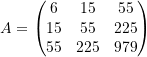8. Calculate the Cholesky Decomposition for the following positive definite symmetric matrix: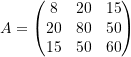Employ the results to find the solution to the system of equations: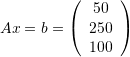9. Find the LU factorization of the following matrix: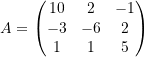Employ the results to find two solutions for the vectorcorresponding to the following two linear systems of equations: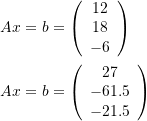10. Using the “Timing” keyword in Mathematica, compare the efficiency of the Cholesky Decomposition code provided above in comparison the built-in “CholeskyDecomposition” function in Mathematica by finding the decomposition for the identity matrix of size. Can you produce a more efficient version of the provided code?
11. Use the “CholeskyDecomposition” built-in function in Mathematica to produce a code to utilize the Cholesky Decomposition method to solve the linear systemwhenis positive definite symmetric matrix. Compare with the Gauss elimination, the Gauss-Jordan elimination and the LU decomposition methods when solving the simple system of equations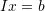whereis a vector whose components are all equal to 1. Similar to problem 4, draw the relationship showing the timing as a function of the size of the matrix.
12. Use Mathematica to program the Jacobi method such that the code would re-arrange the rows of the matrixto ensure that the diagonal entries are all non-zero.
13. Compare the Jacobi method and the Gauss elimination method to solve the simple system of equationswhereis a vector whose components are all equal to 5. Similar to problem 4, draw the relationship showing the timing as a function of the size of the matrix. What do you notice about the Jacobi method in this case?
14. Use the Gauss-Seidel method to find a solution to the following system after converting it into a diagonally dominant system: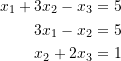15. The following system is not diagonally dominant. Check whether the Jacobi and Gauss-Seidel methods converge or not using an initial guess of the zero vector.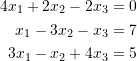16. Assuming a stopping criterion of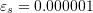, find the optimal value ofin the SOR method producing the fastest convergence in finding the solution to the following system. Compare with the Gauss-Seidel method.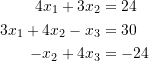17. Use the Gauss-Seidel method with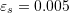to solve the following system of linear equations: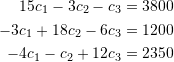18. An electrical engineer supervises the production of three types of electrical components. Three kinds of material: metal, plastic, and rubber are required for production. The amounts needed to produce one unit of component 1 are 15 g of metal, 0.25 g of plastic, and 1.0 g of rubber. The amounts needed to produce one unit of component 2 are 17g of metal, 0.33g of plastic, and 1.2g of rubber. The amounts needed to produce one unit of component 3 are 19 g of metal, 0.42 g of plastic, and 1.6 g of rubber. If a total of 2.12, 0.0434, and 0.164 kg of metal, plastic, and rubber, respectively, are available each day. How many units of each component on average can be produced every day?
19. Use the Gauss-Jordan elimination method to solve the following linear system of equations: